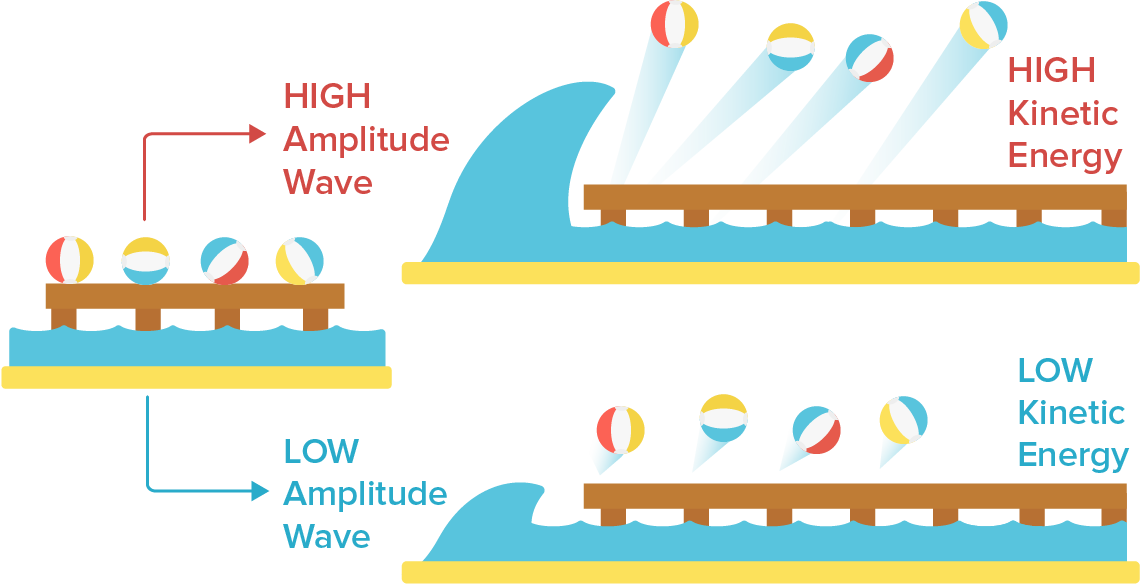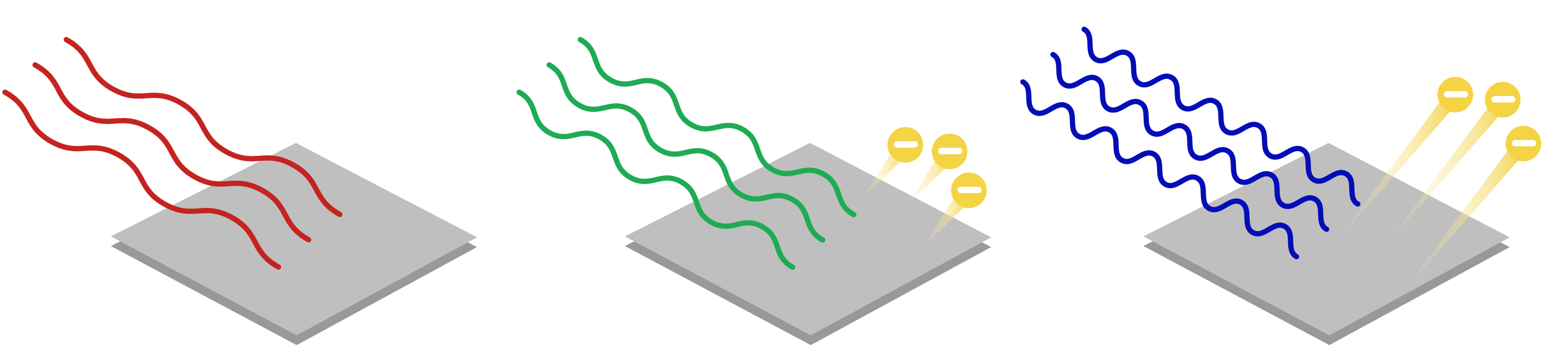# The Origins of Quanta### Science before the Quantum age

Imagine that you were a scientist living in the 1800s. Classical physics, was well understood by everyone. At the time, there are really three branches of physics:

• Classical Mechanics: Newton’s Laws, later to be reformulated by Lagrange and Hamilton. This is where you hear the phrase:

$\sum_i F_i = ma$
• Classical Electrodynamics: The interaction between electric and magnetic fields, circuits, light as electromagnetic radiation, and Maxwell’s Equations.

• Classical Thermodynamics: The study of heat, energy, and systems in equilibrium which started the Industrial revolution.

Scientists believed that all natural phenomenon could be explained by one of these three fields. This description of nature, would be later known as classical physics. Today, we know that classical physics is a limiting case when speeds are very small, and when the size of objects we are talking about is very large.Image: Wikipedia

Classical physics had two differences from quantum theory.

1. Measurement without disturbance. An experimenter would take his equipment and measure the system. And the system would not care about whether the experimenter was there or not. Examples would be the temperature, pressure of a engine. The wavelength of light. The velocity and acceleration of an object.

In the realm of classical mechanics, we can measure these variables to arbitrary precision, as long as our instruments are precise enough.

2. Determinism. Classical physics is based upon deterministic equations. If we know the initial value, we can simply plug the initial data into our equations, and then all future behavior of the system can be predicted.

“We may regard the present state of the universe as the effect of its past and the cause of its future. An intellect which at a certain moment would know all forces that set nature in motion, and all positions of all items of which nature is composed, if this intellect were also vast enough to submit these data to analysis, it would embrace in a single formula the movements of the greatest bodies of the universe and those of the tiniest atom; for such an intellect nothing would be uncertain and the future just like the past would be present before its eyes.”

Pierre Simon Laplace, A Philosophical Essay on Probabilities, (1814)

The uncertainty in classical physics was due to our own ignorance with the instruments. The universe then, was simply an initial value problem. We will explore these ideas of measurement and determinism through the series. For now, let us try to understand the origins of quanta.

### 1905 - The Photoelectric EffectImage: Wikipedia

When a metal surface is illuminated by light, electrons are emitted from the surface. This is the photoelectric effect, which was discovered by Heinrich Rudolph Hertz in 1887 while investigating electromagnetic radiation.

You can think of the photoelectric effect like a solar panel: shine light on a metal to create a current.

The setup is as follows.

• Light is shone on the metal surface, which is called the emitter.
• As a result, electrons are released from the emitter.
• These electrons travel toward the second metal, which is the collector.
• A voltage is applied between the emitter and collector, to register the number of electrons emitted.
• If we do this experiment in a vacuum, we can determine the rate of the electrons emitted, the current $i$, and the maximum kinetic energy, $K_{max}$ by tuning the voltage.

If the electrons have less than the maximum kinetic energy, the voltage will stop them from passing. Otherwise, we can determine the voltage known as the stopping potential that will stop all but the most energetic electrons, with the equation

$K_{max} = eV_{stop}$

#### Classical Analysis

Let us first try to solve the problem according to classical physics. According to classical electromagnetism, light was a electromagnetic wave composed of oscillating electric and magnetic fields.

• The metal surface is illuminated with light of intensity $I$.
• The electron on the surface absorbs energy continuously from the wave until the electron has enough energy to overcome the binding energy of the metal.
• This minimum value of energy required to free an electron from the surface is called the work function or binding energy.

Classical theory then predicts:

1. The maximum kinetic energy of the photoelectrons is proportional to the intensity of light, which is proportional to a wave’s amplitude.

The intensity of light is just the brightness, and so you would intuitively expect as the light is brighter the light would carry more energy. Intensity is related to the amplitude (electric field) of a electromagnetic wave by:

$I = \frac{\epsilon_0 c |\mathbf{\vec{E}}|^2}{2}$

Classical theory predicts as the intensity of the light is increased, more energy is given to the surface and the emitted photoelectrons are released with more energy. Since $I$ increases, the resulting electric field $\mathbf{\vec{E}}$ must increase, and so there is an increased force on the electron:

$F = -e\mathbf{\vec{E}}$

and so there is an increased kinetic energy when it is emitted. Consider the following graphic as an analogy:2. The photoelectric effect should occur for light of any frequency. As long as the intensity $I$ is large enough, the electrons should be emitted.

3. There should be a delay after the light hits the surface. To estimate the time required, suppose the atom has surface area $\pi r^2$ and has binding energy $E_{bind}$. Since intensity has units of $\mathrm{W/m^2}$, which is power/surface area. Power has units of energy*time, and rearranging, we solve for the time taken which is

$\Delta t=\frac{E_{\mathrm{bind}}}{I \pi r^{2}}$

For $I = 120 \mathrm{W/m^2}$, (helium-neon laser), $E_{bind} = 4 \mathrm{eV}$ (binding energy of Aluminum), and assuming the atoms has $r = 0.1 \mathrm{nm}$, the time is approximately $0.2$ seconds.

#### Experimental Observations

The experimental observations were well known by 1902. However:

1. The maximum kinetic energy is completely independent of the intensity of incident radiation, and is instead related to a wave’s frequency, not amplitude. Doubling the intensity does not change the stopping potential, which means that the kinetic energy of the electron is not affected by intensity. This greatly contradicts the classical prediction.2. Photoelectrons are not emitted for light of any frequency. The light must have a minimum frequency for the photoelectric effect to be observed. The value of this frequency is called the cutoff frequency $f_c$. For light with frequency less than $f_c$, no photoelectric effect is observed, and no electrons are ejected. This also greatly contradicts the classical prediction.

3. The photoelectrons are emitted almost instantaneously, rather than having a delay.

#### Einstein’s Solution

Einstein based his solution off of Max Planck, which used the idea of quanta to solve his problem of Blackbody Radiation, which we will cover later. Einstein suggested that light was not a continuous wave, but a wave packet called a photon. Photons came in discrete wave packets called quanta. Indeed, it was because light was composed of quanta that allowed it to behave like particles. This would resolve the photoelectric effect: One photon in, one electron out.

To fit the experimental data, the energy of a photon would have frequency $f$, and a proportionality constant called Planck’s constant $h$:

$E = hf = \frac{h c}{\lambda}$

We can write this in terms of the wavelength with the universal wave equation $v = f\lambda$, where $c$ is the speed of light, and $\lambda$ is the wavelength.

The explanation of the photoelectric effect is:

• A photoelectron is a result of the absorption of a single photon. The photons energy is transferred instantaneously.

• If the photon energy $hf$ is greater than the binding energy $E_{bind}$, an electron is released with kinetic energy

$K_{max }=hf-E_{bind}$

Notice that the kinetic energy does not depend on the intensity of light.

• Doubling the intensity means that twice as many photons are emitted, but they have the same maximum kinetic energy.

To experimentally test this, we write use the stopping potential to write

$V_{s}=\frac{h f}{e}-\frac{E_{bind}}{e}$

If we look at stopping potential $V_s$ and frequency $f$, since $E_{bind}/e$ is a constant we expect the relation to be linear. From this, we can calculate the slope of the graph and hence the value of Planck’s constant.

Robert Millikan obtained a value of $h=6.57 \times 10^{-34} \mathrm{Js}$, which is 1% away from the currently accepted value of Planck’s constant $h = 6.626 \times 10^{-34} \mathrm{Js}$.

Millikan was awarded the 1923 Nobel Prize in physics for his careful experiments on the photoelectric effect, and Einstein was awarded the 1921 Nobel Prize in physics for his explanation of the photoelectric effect.

The explanation given by Einstein seemed mysterious. Light had to be a single particle in order to explain the photoelectric effect. Yet at the same time, light had to be an electromagnetic wave to agree with classical electromagnetism. How was it then, that light could be a particle and a wave at the same time?

Khan Academy has a fantastic resource on the photoelectric effect: The photoelectric effect on Khan Academy

One of my favourite Youtubers, Viascience also made an excellent video on the topic: